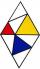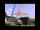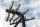# Diamond diagonals

Calculate the diamonds' diagonals lengths if the diamond area is 156 cm square and the side length is 13 cm.

Result

u =  21.633 cm
v =  14.422 cm

#### Solution:Checkout calculation with our calculator of quadratic equations.Leave us a comment of example and its solution (i.e. if it is still somewhat unclear...):

Showing 0 comments:Be the first to comment!#### To solve this verbal math problem are needed these knowledge from mathematics:

Looking for help with calculating roots of a quadratic equation? Do you have a linear equation or system of equations and looking for its solution? Or do you have quadratic equation? Pythagorean theorem is the base for the right triangle calculator.

## Next similar examples:

1. Diamond and diagonalsA diamond has diagonals f = 8 cm and g = 6 cm long. How long is this diamond perimeter? (Calculate it!)
2. Diamond diagonalsFind the diamond diagonal's lengths if the area is 156 cm2 and side is 13 cm long.
3. Diagonals of diamondFind the area and circumference of the diamond ABCD with 15m and 11m diagonals.
4. ParkIn the park is marked diamond shaped line connecting locations A, D, S, C, B, A. Calculate its length if |AB| = 108 m, |AC| = 172.8 m.
5. Diagonals in diamons/rhombusRhombus ABCD has side length AB = 4 cm and a length of one diagonal of 6.4 cm. Calculate the length of the other diagonal.
6. Diagonals of a rhombusOne of the diagonals of a rhombus is twice as the other. If the sum of the lengths of the diagonals is 24, find the area of the rhombus.
7. RhombusThe rhombus has diagonal lengths of 4.2cm and 3.4cm. Calculate the length of the sides of the rhombus and its height
8. Diagonal to areaCalculate the area of a rectangle in which the length of the diagonal is 10 cm.
9. Square diagonalCalculate the length of diagonal of the square with side a = 23 cm.
10. Equilateral triangleThe equilateral triangle has a 23 cm long side. Calculate its content area.
11. TriangleCalculate the sides of the triangle if its area S = 630 and the second cathethus is shorter by 17.
12. Rhombus diagonalArea of rhombus is 224. One diagonal measures 33, find length of other diagonal.
13. SatinSanusha buys a piece of satin 2.4 m wide. The diagonal length of the fabric is 4m. What is the length of the piece of satin?
14. A mastA mast 32 meters high was broken by the wind so that its top touches the ground 16 meters from the pole. The still standing part of the mast, the broken part and the ground form a rectangular triangle. At what height was the mast broken?
15. Holidays - on poolChildren's tickets to the swimming pool stands x € for an adult is € 2 more expensive. There was m children in the swimming pool and adults three times less. How many euros make treasurer for pool entry?
16. Fifth of the numberThe fifth of the number is by 24 less than that number. What is the number?
17. ColumnPerpendicular pole high 8 m tall broke and its toe fell 2.7 m from the bottom of the pole. At what height above the ground pole broke?